Ex 11.3

Chapter 11 Class 12 Three Dimensional Geometry
Serial order wise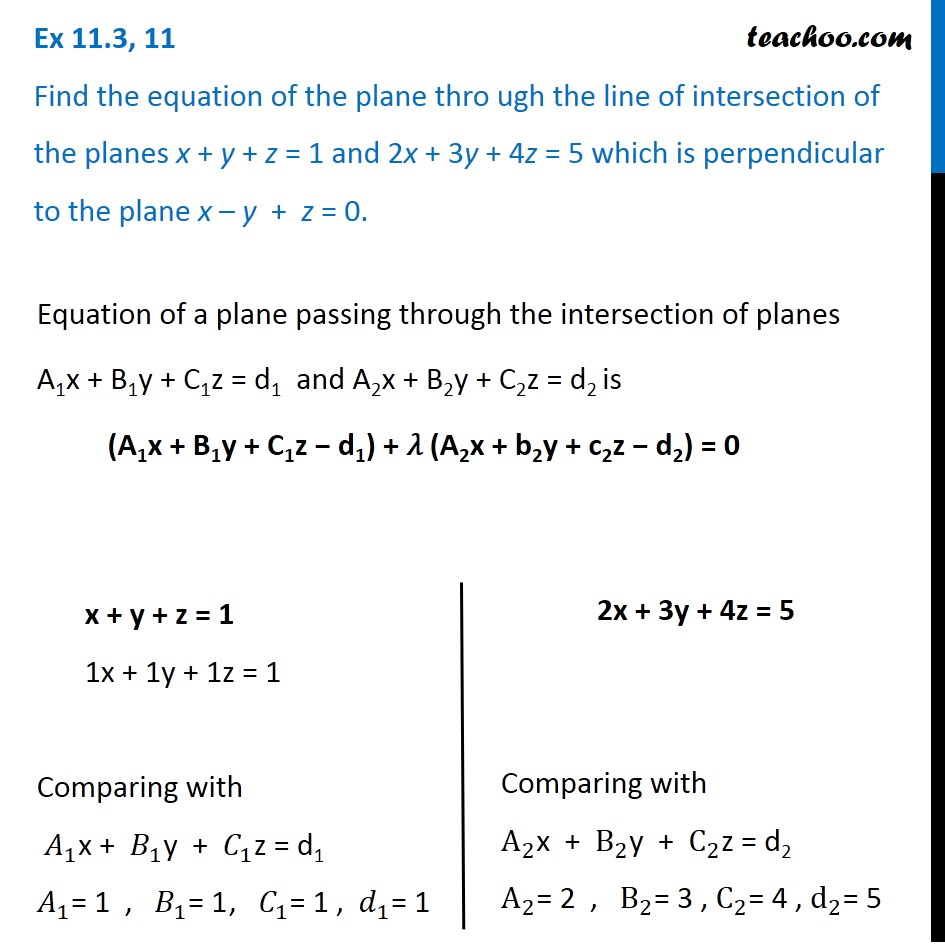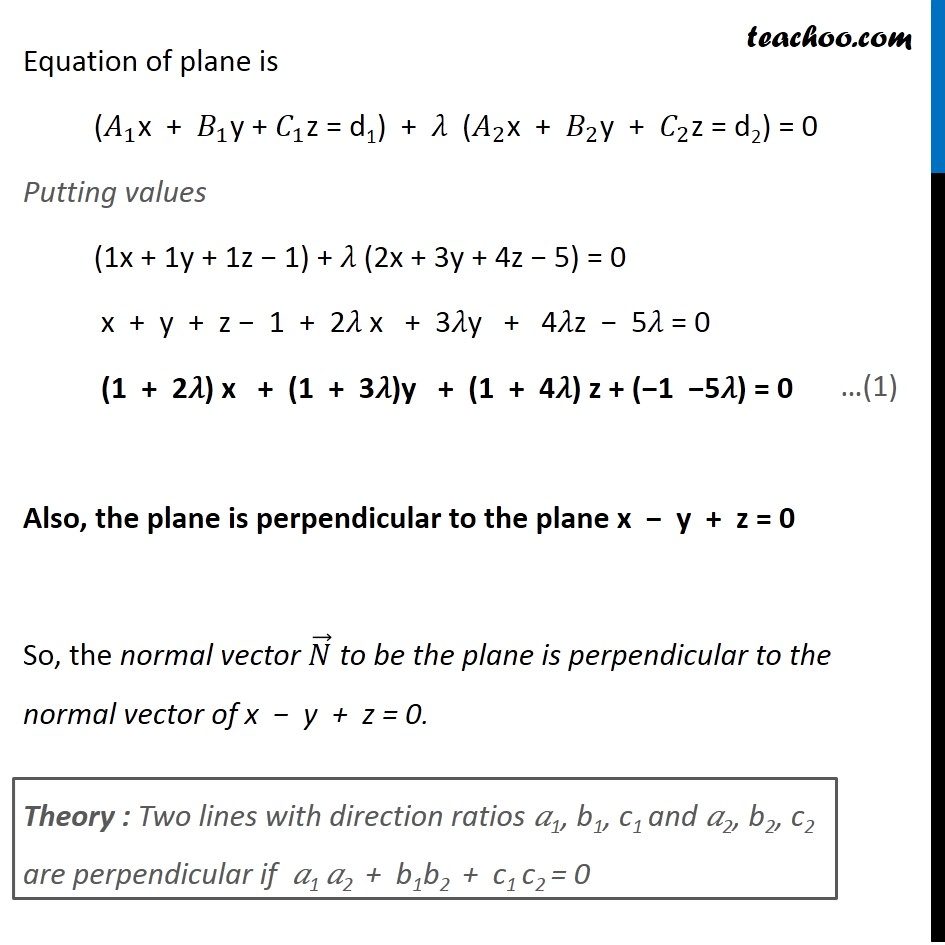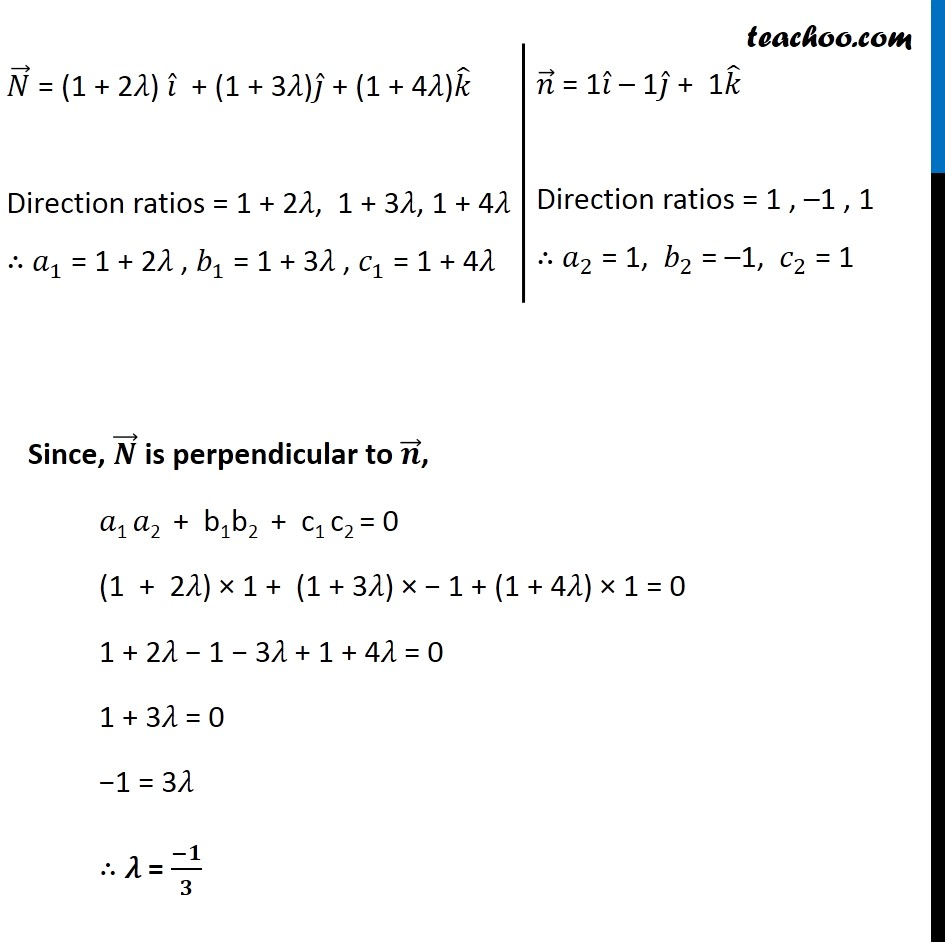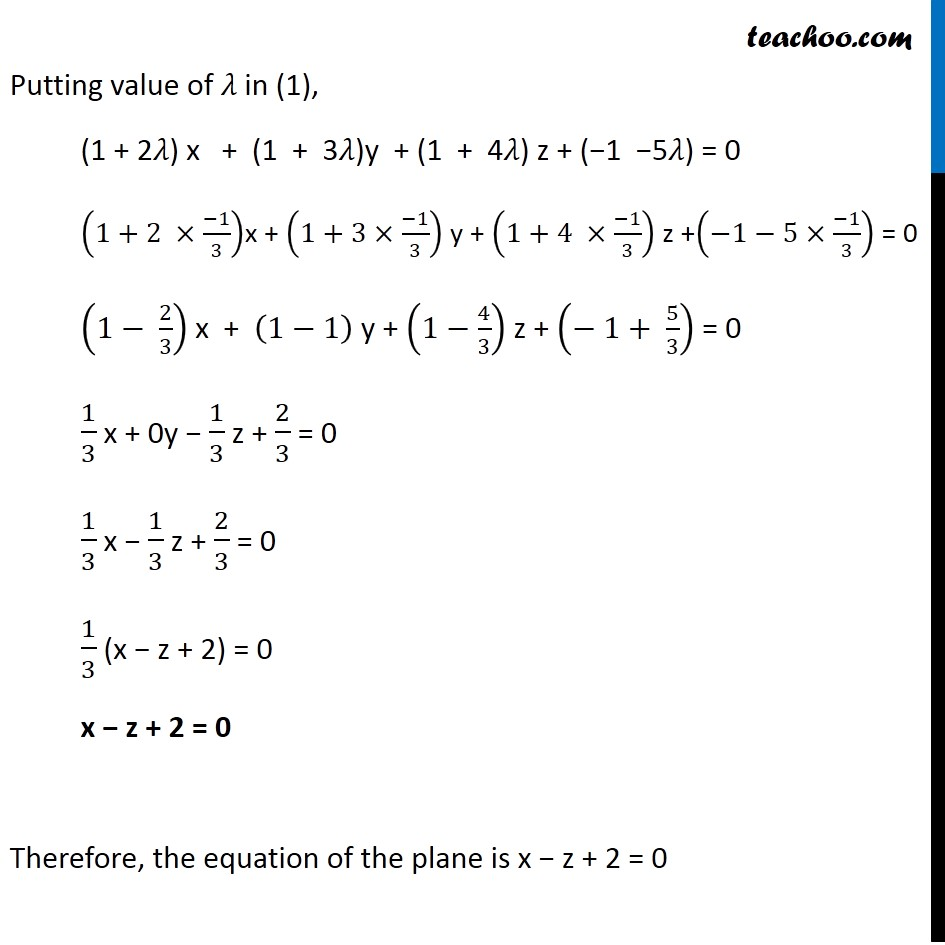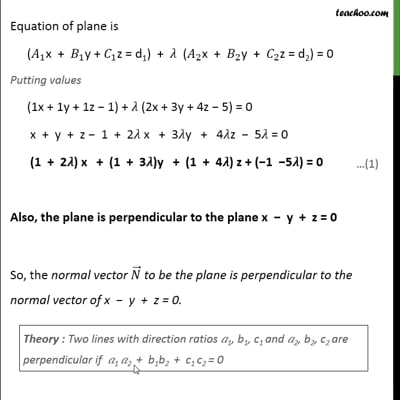This video is only available for Teachoo black users

Solve all your doubts with Teachoo Black (new monthly pack available now!)

### Transcript

Ex 11.3, 11 Find the equation of the plane thro ugh the line of intersection of the planes x + y + z = 1 and 2x + 3y + 4z = 5 which is perpendicular to the plane x – y + z = 0.Equation of a plane passing through the intersection of planes A1x + B1y + C1z = d1 and A2x + B2y + C2z = d2 is (A1x + B1y + C1z − d1) + 𝜆 (A2x + b2y + c2z − d2) = 0 x + y + z = 1 1x + 1y + 1z = 1 Comparing with 𝐴_1 "x" + 𝐵_1 "y" + 𝐶_1 "z" = d1 𝐴_1= 1 , 𝐵_1= 1 , 𝐶_1= 1 , 𝑑_1= 1 2x + 3y + 4z = 5 Comparing with A_2 "x" + B_2 "y" + C_2 "z" = d2 A_2= 2 , B_2= 3 , C_2= 4 , d_2= 5 Equation of plane is (𝐴_1 "x" + 𝐵_1 "y" + 𝐶_1 "z" = d1) + 𝜆 (𝐴_2 "x" + 𝐵_2 "y" + 𝐶_2 "z" = d2) = 0 Putting values (1x + 1y + 1z − 1) + 𝜆 (2x + 3y + 4z − 5) = 0 x + y + z − 1 + 2𝜆 x + 3𝜆y + 4𝜆z − 5𝜆 = 0 (1 + 2𝜆) x + (1 + 3𝜆)y + (1 + 4𝜆) z + (−1 −5𝜆) = 0 Also, the plane is perpendicular to the plane x − y + z = 0 So, the normal vector 𝑁 ⃗ to be the plane is perpendicular to the normal vector of x − y + z = 0. Theory : Two lines with direction ratios 𝑎1, b1, c1 and 𝑎2, b2, c2 are perpendicular if 𝑎1 𝑎2 + b1b2 + c1 c2 = 0 𝑁 ⃗ = (1 + 2𝜆) 𝑖 ̂ + (1 + 3𝜆)𝑗 ̂ + (1 + 4𝜆)𝑘 ̂ Direction ratios = 1 + 2𝜆, 1 + 3𝜆, 1 + 4𝜆 ∴ 𝑎_1 = 1 + 2𝜆 , 𝑏_1 = 1 + 3𝜆 , 𝑐_1 = 1 + 4𝜆 𝑛 ⃗ = 1𝑖 ̂ – 1𝑗 ̂ + 1𝑘 ̂ Direction ratios = 1 , –1 , 1 ∴ 𝑎_2 = 1, 𝑏_2 = –1, 𝑐_2 = 1 Since, 𝑵 ⃗ is perpendicular to 𝒏 ⃗, 𝑎1 𝑎2 + b1b2 + c1 c2 = 0 (1 + 2𝜆) × 1 + (1 + 3𝜆) × − 1 + (1 + 4𝜆) × 1 = 0 1 + 2𝜆 − 1 − 3𝜆 + 1 + 4𝜆 = 0 1 + 3𝜆 = 0 −1 = 3𝜆 ∴ 𝜆 = (−𝟏)/𝟑 Putting value of 𝜆 in (1), (1 + 2𝜆) x + (1 + 3𝜆)y + (1 + 4𝜆) z + (−1 −5𝜆) = 0 (1+2 ×(−1)/3)x + (1+3×(−1)/3) y + (1+4 ×(−1)/3) z +(−1−5×(−1)/3) = 0 (1− 2/3) x + (1−1) y + (1−4/3) z + (− 1+ 5/3) = 0 1/3 x + 0y − 1/3 z + 2/3 = 0 1/3 x − 1/3 z + 2/3 = 0 1/3 (x − z + 2) = 0 x − z + 2 = 0 Therefore, the equation of the plane is x − z + 2 = 0